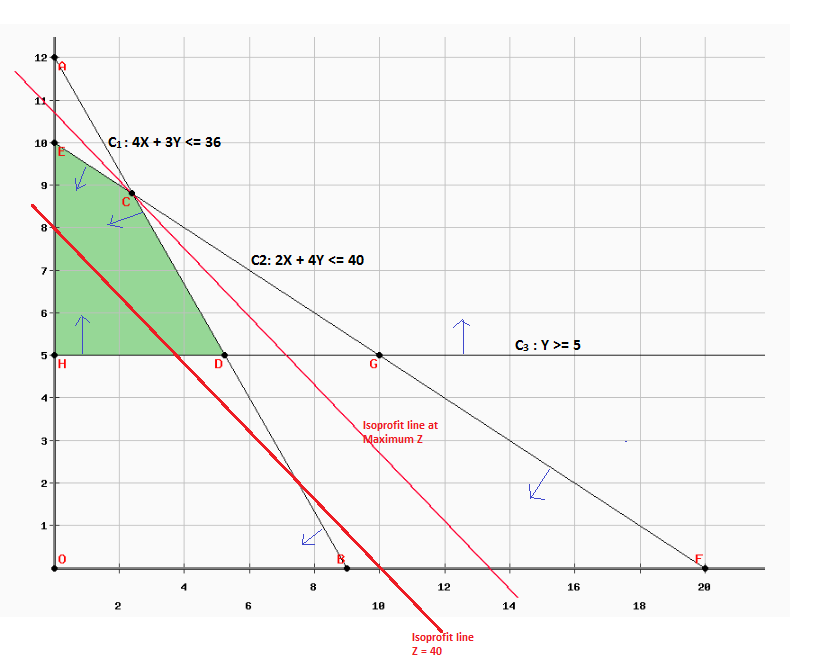# Solved: LP. Model: Maximize Z = 4X +5Y Subject to: 4X +3Y lessthanorequalto 36 (C_1) 2x + 4Y lessthanorequalto 40 (C_2) 1Y greaterthanorequalto 5 (C_3) X, Y greaterthanorequalto 0 Plot and label the constraints C_1, C_2 and C_3 (using

LP. Model: Maximize Z = 4X +5Y Subject to: 4X +3Y lessthanorequalto 36 (C_1) 2x + 4Y lessthanorequalto 40 (C_2) 1Y greaterthanorequalto 5 (C_3) X, Y greaterthanorequalto 0 Plot and label the constraints C_1, C_2 and C_3 (using the line drawing tool) on the provided graph. Using the point drawing tool, plot the point that maximizes the objective function.

Maximize Z = 4X + 5Y

Subject To:

Don't use plagiarized sources. Get Your Custom Essay on
Solved: LP. Model: Maximize Z = 4X +5Y Subject to: 4X +3Y lessthanorequalto 36 (C_1) 2x + 4Y lessthanorequalto 40 (C_2) 1Y greaterthanorequalto 5 (C_3) X, Y greaterthanorequalto 0 Plot and label the constraints C_1, C_2 and C_3 (using
GET AN ESSAY WRITTEN FOR YOU FROM AS LOW AS \$13/PAGE

4X + 3Y <= 36           (C1)

2X + 4Y <= 40           (C2)

Y >= 5                      (C3)

X, Y >= 0

Graphical Solution:

Plotting of constraints on graph:

Convert the inequalities in the equality equation and find the X-axis and Y-axis intercepts to plot the equation lines.

 Sr. No. Constraints X-axis intercept (When x2 = 0) Y-axis intercept (When x1 = 0) C1 4X + 3Y = 36 B (9, 0) A (0, 12) 2X + 4Y = 40 F (20, 0) E (0, 10) Y = 5 Parallel to X-axis H (0, 5)The graph is shown below. The arrows for constraint lines determine the feasible area for the respective constraints. The shaded region (GREEN) represents feasible region which satisfies all the constraints. The feasible region is HECD.

Now plot Iso-profit line for profit of \$40, the x and Y coordinate is:

4X + 5Y = 40

When y = 0, X = 10, X-axis Coordinate is (10, 0)

When X = 0, y = 8, Y-axis Coordinate is (0, 8)

Draw line parallel to Iso-profit line Z = 40, so that it meets the feasible region at the highest point. The highest point intersection is at point C. Thus, the objective function value is maximized t point C.

Coordinate of Point C:

Point C is intersection point of Constraint C1 and C2.

Operation: (2 x C2) – C1

2 x (2X + 4Y) – 4X – 3Y = 2 x 40 – 36

4X + 8Y – 4X – 3Y = 44

5Y = 44

Y = 8.8

Substitute Y = 8.8 in equation C1

4X + 3(8.8) = 36

4X + 26.4 = 36

X = (36 – 26.4)/4 = 2.4

Coordinates of C: (2.4, 8.8)

Profit at Point C = 4X + 5Y = (4)(2.4) + (5)(8.8) = 9.6 + 44

Profit at Point C =53.6

Since at the point C, the objective function is maximized, Optimal Z = 53.6 where

X = 2.4 and Y = 8.8